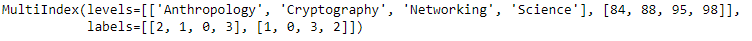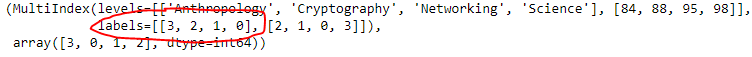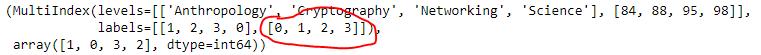# Python | Pandas MultiIndex.sortlevel()

• Last Updated : 24 Dec, 2018

Python is a great language for doing data analysis, primarily because of the fantastic ecosystem of data-centric python packages. Pandas is one of those packages and makes importing and analyzing data much easier.

Pandas` MultiIndex.sortlevel()` function sort MultiIndex at the requested level. The result will respect the original ordering of the associated factor at that level.

Syntax: MultiIndex.sortlevel(level=0, ascending=True, sort_remaining=True)

Parameters :
level : [list-like, int or str, default 0] If a string is given, must be a name of the level If list-like must be names or ints of levels
ascending : False to sort in descending order Can also be a list to specify a directed ordering
sort_remaining : sort by the remaining levels after level.

Returns :
sorted_index : Resulting index
indexer : Indices of output values in original index

Example #1: Use `MultiIndex.sortlevel()` function to sort the 0th level of the MultiIndex in descending order.

 `# importing pandas as pd``import` `pandas as pd`` ` `# Create the MultiIndex``midx ``=` `pd.MultiIndex.from_arrays([[``'Networking'``, ``'Cryptography'``, ``                                     ``'Anthropology'``, ``'Science'``],``                                             ``[``88``, ``84``, ``98``, ``95``]])`` ` `# Print the MultiIndex``print``(midx)`

Output :Now let’s sort the 0th level of the MultiIndex in descending order.

 `# sort the 0th level in descending order.``midx.sortlevel(level ``=` `0``, ascending ``=` `False``)`

Output :As we can see in the output, the function has returned a new object having the 0th level sorted in descending order.

Example #2: Use `MultiIndex.sortlevel()` function to sort the 1st level of the MultiIndex in the increasing order.

 `# importing pandas as pd``import` `pandas as pd`` ` `# Create the MultiIndex``midx ``=` `pd.MultiIndex.from_arrays([[``'Networking'``, ``'Cryptography'``, ``                                     ``'Anthropology'``, ``'Science'``], ``                                             ``[``88``, ``84``, ``98``, ``95``]])`` ` `# Print the MultiIndex``print``(midx)`

Output :Now let’s sort the 1st level of the MultiIndex in increasing order.

 `# sort the 1st level of the MultiIndex in increasing order.``midx.sortlevel(level ``=` `1``, ascending ``=` `True``)`

Output :As we can see in the output, the function has returned a new object having the first level sorted in increasing order.

My Personal Notes arrow_drop_up